# XL abc's

(redirected from Excel tutorial)

## XL abc's

This tutorial is for newcomers to spreadsheets. The examples apply to all versions of Excel, whether Windows or Mac, as well as to non-Microsoft spreadsheets.

The topics covered in XL abc's are replicated under their own names with an XL prefix. For example, to review later how to delete rows or columns, look up XL Delete row/column.

Spreadsheets display a matrix or rows and columns, the intersections of which are called "cells." The three primary things a cell can contain are:

1. LABELS - Descriptive text.

2. VALUES - Numeric data.

3. FORMULAS - An algorithm that states how data in the cells will be calculated. Functions are predefined formulas such as SUM, which adds numbers in a row or column.

### Workbooks and Worksheets

A document created in Excel is called a "workbook," and workbooks can include any number of pages, called "worksheets." Each worksheet is like having another document but enables related data to be kept in one file.

When you create a new blank document, it starts out with three blank worksheets, and more can be added (see XL Adding worksheets). Excel workbooks are saved with an .XLS or .XLSX extension.

Create a Workbook
When Excel is launched, a pre-formatted template can be selected for various purposes such as budgets, calendars and receipts. If "blank document" is selected, a matrix of empty rows and columns is created. New "blank documents" are named Book1 or Workbook1, while documents using pre-formatted templates generally use the template name, such as ExpenseReport1 and SalesBudget1. All documents are easily renamed when saved.

One Workbook - Three Worksheets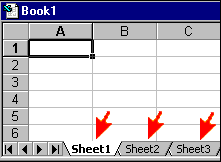Worksheets are selected by clicking the tabs at the bottom of the window. They can be renamed or deleted, and new worksheets can be added by right clicking the tab and selecting from the menu.

Documents Are Sequentially Numbered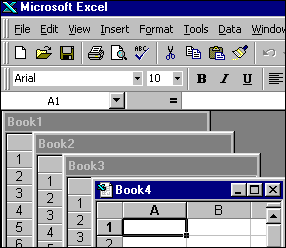If more than one workbook is created in the same Excel session, Excel names them Book1, Book2 (or SalesExpense1, SalesExpense2) and so on.

### File Management and Exit

Following are the essential file management functions in Excel.

Open
To open an Excel workbook, select File/Open and the name of the workbook.

Save
To save a workbook, select File/Save. The first time a workbook is saved, it can be renamed by typing over the default name. When finished with the workbook, select File/Close.

Make Copies - Save As
Select File/Save As to copy the workbook to a different folder or to make a copy with a different name. Save As always asks for a file name. If you never changed the default file name, Save will also prompt you for a name. However, once you rename it, Save will save changes without prompting.

Exit
Select File/Exit or click the X in the upper right corner. If changes were made, Excel prompts you to save them.

File Functions in the File Menu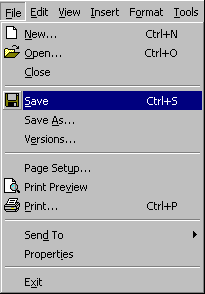All file management options are selected in the File menu. In the Mac, to exit, select Excel/Quit Excel.

### Rows, Columns and Cells

Worksheets are made up of cells organized in rows and columns. The height of rows and the width of columns can be changed (see below), and rows and columns can be added and deleted (see XL Insert row/column and XL Delete row/column).

At any given time, only one cell is active. The active cell has a bold border, and its row and column headers are also bold (in the following example, A1 is the active cell).

To enter descriptive text (labels) and numbers (values), select a cell and start typing. When done, press Enter, and the active cell moves to the one below.

Format Options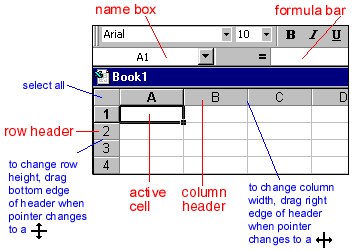The icons (top) are either on a toolbar or are displayed by right clicking the cells and selecting Format/Cells.

### Labels and Values

Labels are descriptive text, and values are quantities, money amounts and dates. Labels and values only change by typing new data on top of the old.

Labels
If the label starts with a letter, just type it. If it starts with anumeric digit, type an apostrophe first. The apostrophe tells Excel that the numeric data that follows is not a value, but a descriptive label. In the following examples, "Grades for Semester" is just typed into the cell, but the 115 for Model # must be typed with an apostrophe first ('115) to define it as a label and not a number that can be calculated.

Format Options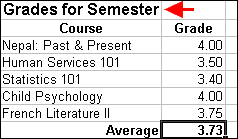The icons (top) are either on a toolbar or are displayed by right clicking the cells and selecting Format/Cells.

Format Options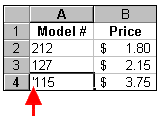The icons (top) are either on a toolbar or are displayed by right clicking the cells and selecting Format/Cells.

Values
Values are numeric data used in calculations, but they can also be dates and times. Values are only changed by the user. In the examples above, the numbers under Grade and Price are values. The dollar signs are displayed automatically because the cell is formatted as a money amount.

Formatting
There are numerous options for formatting text and numeric values (font style, alignment, dollar signs, commas, etc.). Select one or more cells to apply a format and right click to retrieve the menu. Select Format/Cells. The Special category (under Number) provides formats for zip code, phone number and social security number.

Format Options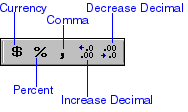The icons (top) are either on a toolbar or are displayed by right clicking the cells and selecting Format/Cells.

Format Options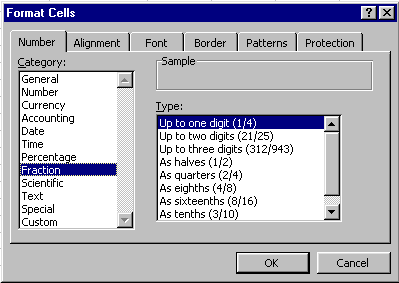The icons (top) are either on a toolbar or are displayed by right clicking the cells and selecting Format/Cells.

### Formulas and Functions

Formulas provide the "ripple through" effect that occurs when you make a change to any of the numeric values in the cells, and all the formulas are recalculated automatically. A formula is an algorithm, or mathematical expression, that says "add this to that" or "sum this column and multiply by 5." A function is a predefined formula that saves steps. For example, Sum is a function that adds all the numbers in contiguous vertical or horizontal cells. Following are the math operators used in formulas:

```   +  Addition
-  Subtraction
*  Multiplication
/  Division
%  Percent
( and )  Parentheses group items together
```

Creating and Editing Formulas
Formulas are created by selecting cells and pressing the appropriate math keys on the keyboard or function buttons. You create a sequence of events such as "this cell minus that cell divided by that cell."

After formulas have been created, you can edit them manually. When you select a cell that contains a formula, the formula is visible in the Formula Bar, where it is available for editing. In the following example, the contents of B2 are subtracted from B1. An equal sign is always the first character of a formula.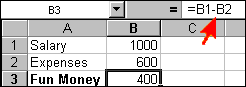Adding (Summing) Columns and Rows
To add a column or row of numbers, select the empty cell under the column or on the right side of the row, click the SUM button in the Formulas ribbon (or on the toolbar in older Excel versions), then press Enter. The SUM function will add a column or a row of numbers.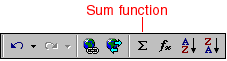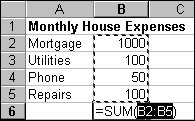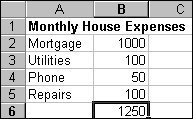Subtracting Numbers
To subtract one number from another, do the following steps. See example below:

1. Select the cell that will hold the result.
2. Press equal key.
3. Select the cell you want to subtract from.
4. Press subtract key (- key).
5. Select the cell to be subtracted.
6. Press Enter key.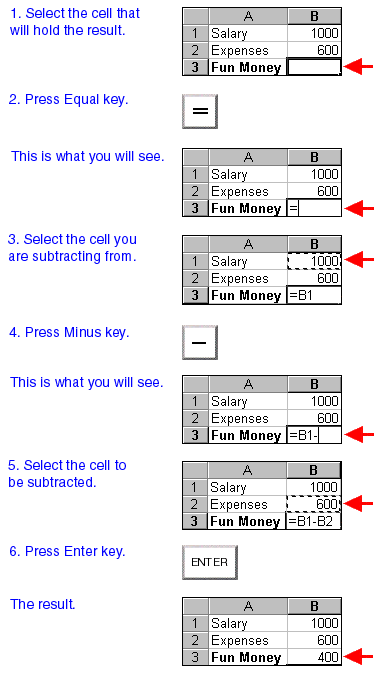Multiplying Numbers
To multiply one number with another, do the following steps. An example is provided below:

1. Select the cell that will hold the result.
2. Press equal key
3. Select the cell you want to multiply.
4. Press asterisk key (* key).
5. Select the cell with the multiplier.
6. Press Enter key.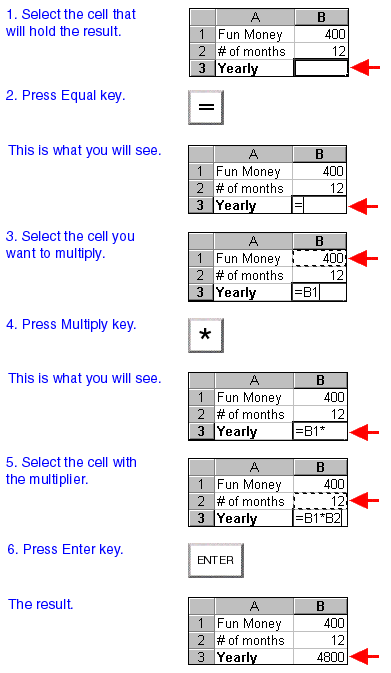Dividing Numbers
To divide one number with another, do the following steps. An example is provided below:

1. Select the cell that will hold the result.
2. Press equal key.
3. Select the cell containing the numerator.
4. Press divide key.
5. Select the cell with the denominator.
6. Press Enter key.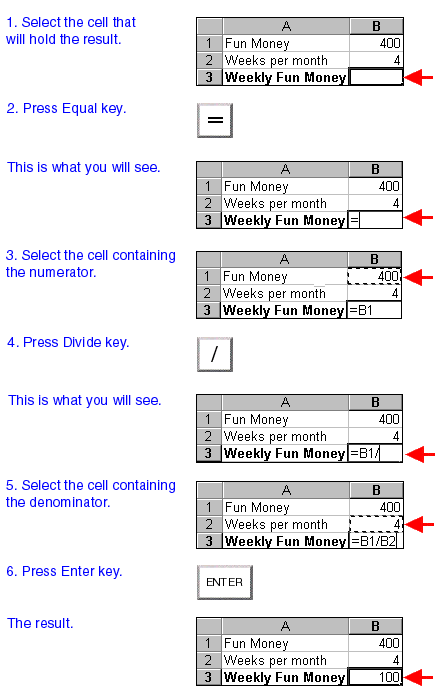Compound Operations and Parentheses
Very often, there is a need to combine two or more mathematical operations in the same formula. When you do that, you often need to use parentheses to group the calculations in the order you want them to be executed. Excel uses standard algebraic rules to perform operations in the following order: (1) exponentiation, (2) multiplication and division and (3) addition and subtraction. However, Excel will always perform operations grouped in parentheses first.

The following example diagrams the steps in developing a compound operation. It calculates gross pay from hours worked in a week which includes regular pay, overtime at time and a half and double time. The formula being built is hourly rate times all the hours worked, but the hours worked are grouped in parentheses so they are treated as a unit and added together. At the end is another example that shows what you would get if you do not use parentheses. The formula in this example is:

gross pay = hourly rate X hours worked

hours worked is computed by

(regular hours +

1.5 X overtime hours +

2 X double time hours)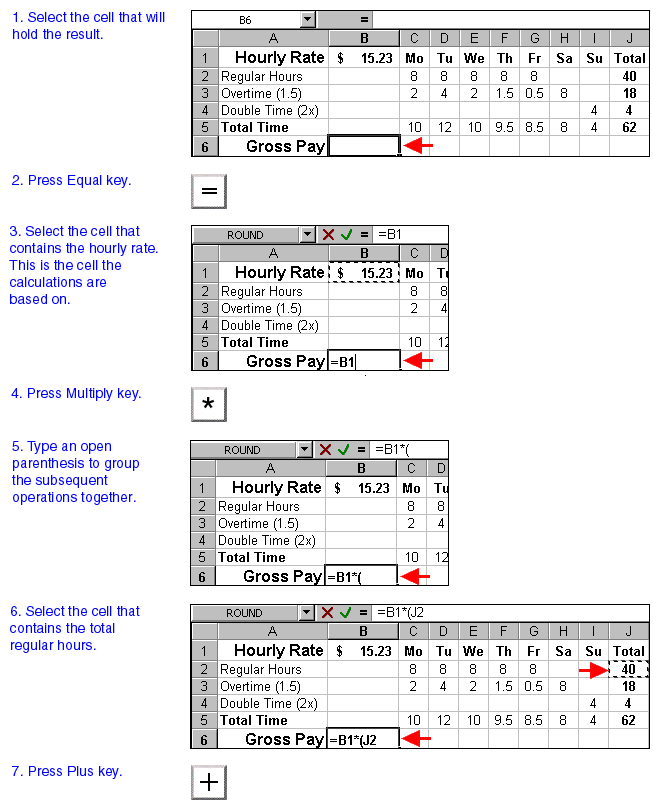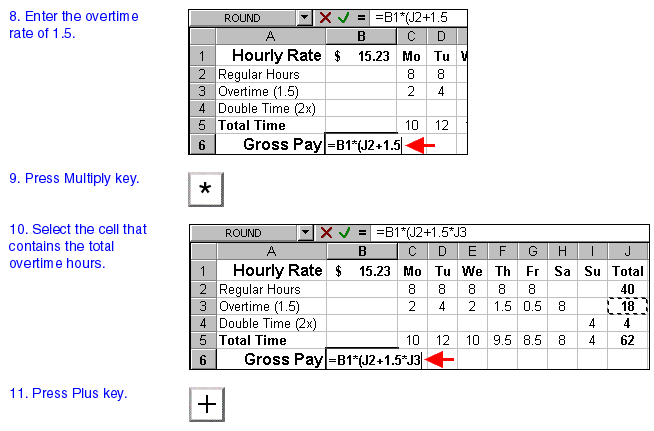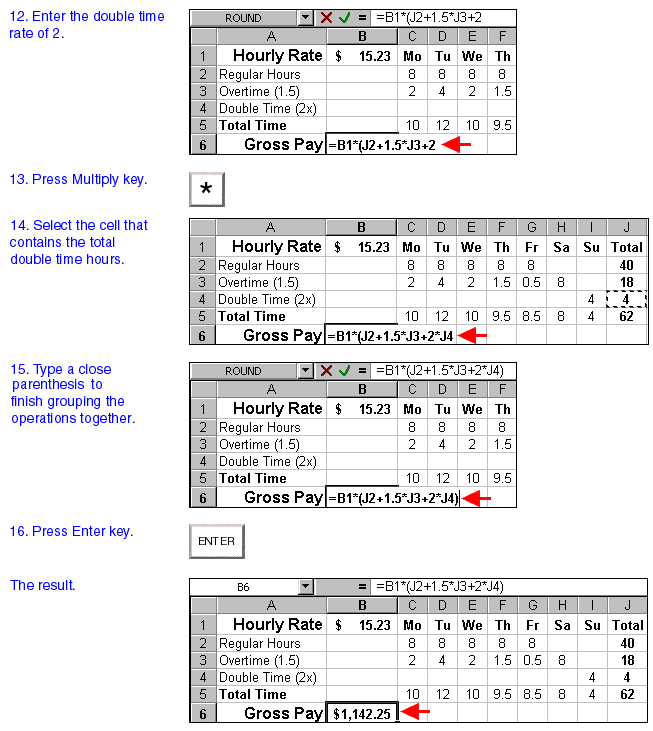You Need the Parentheses
If you hadn't used parentheses in the formula, you would have gotten the wrong results. With parentheses correctly used, the multiplications and additions within the parentheses were computed first. Then the rate was multiplied by that result in the following order:

1. 1.5 x 18 hours = 27 hours
2. 2 x 4 hours = 8 hours
3. 40 + 27 + 8 = 75 hours
4. \$15.23 x 75 hours = \$1142.25

Without parentheses, the dollar payment for the first 40 hours is incorrectly added to the hours worked as follows:

1. \$15.23 x 40 hours = \$609.20
2. 1.5 x 18 hours = 27 hours
3. 2 x 4 hours = 8 hours
4. \$609.20 + 27 + 8 = \$644.20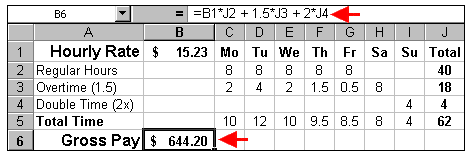Copyright © 1981-2019 by The Computer Language Company Inc. All Rights reserved. THIS DEFINITION IS FOR PERSONAL USE ONLY. All other reproduction is strictly prohibited without permission from the publisher.
Mentioned in ?
References in periodicals archive ?
Additionally, I've had success searching with such key phrases "excel tutorial to develop median price" and "using excel to make graphs with MLS data."
The CD-ROM contains an Excel tutorial and working papers.
(1.) Excel for Science and Technology by Peter Gaeng (Grand Rapids: Abacus, 1993) is partly a brief Excel tutorial but mainly an instruction manual on using Excel in a number of disciplines including mathematics, the social sciences, and financial management.
“Our previous Excel tutorials show learners the basics of writing formulas, but formulas can still be intimidating.

Site: Follow: Share:
Open / Close